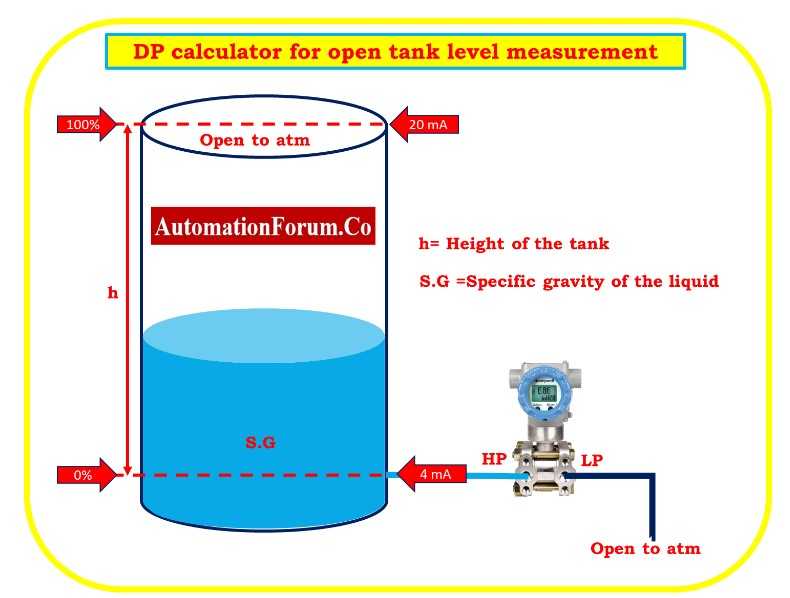# DP calculator for open tank level measurementsBasic level measurement is done using open tank level. Its calculation is relatively easy and is shown below.

## Zero based direct mount application

The level transmitter is positioned in the same level position as the HP tapping point when it is connected.

Pressure on H.P. side of transmitter  P.HP = Patm + (SG*h)

Pressure on LP side of the transmitter P.LP= Patm(open to atmospheric pressure)

The transmitter’s differential pressure is calculated as follows:

DP = P.HP – P.LP

DP = Patm + (SG*h) – Patm

DP   = SG*h

## LRV Calculation

In order to obtain LRV, the pressure on the HP side and LP side of the tank must be empty. When the tank is empty, there will be no pressure on either the HP side or the LP side. since there will be no liquid height.

So h = 0

Then  DP = Patm+(SG*h) – Patm

PH.P = Patm + (SG*0)

=Patm

PL.P = Patm

LRV =  PH.P. –  PL.P

= Patm – Patm

LRV=0

## Estimating the URV

When the tank is completely full of liquid (h), determine the transmitter’s differential pressure (DP).

PH.P  = Patm +(SG*h)

PL.P  = Patm

URV  =  P.HP –  P.LP

= Patm+(S.G*h) – Patm

URV = S.G*h

Range of the transmitter =  0 to (S.G*h)